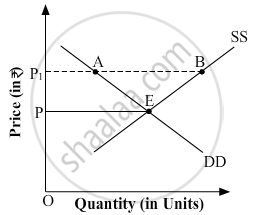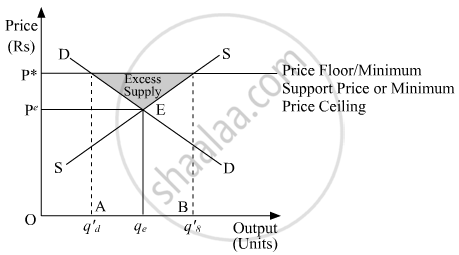# Answer the Following Question. in the Given Diagram, Op is the Market-determined Price, and Op1 Is the Price Fixed by the Government. - Economics

In the given diagram, OP is the market-determined price, and OP1 is the price fixed by the government.(a) Identify if the diagram represents, price ceiling or price flooring.
(b) Discuss the likely behaviour of the market in the given condition.

#### Solution

(a) The diagram represents price flooring.

(b) The price floor implies legislated or government fixed minimum price that should be charged by the seller. The minimum price is fixed above the equilibrium price. In the following figure, DD represents the market demand and SS represents the market supply. The point 'E' represents the market equilibrium point, where the market demand and market supply intersect. The equilibrium price is OPe and equilibrium output is Oqe.

Now, assume that the government imposes the price floor at price OP1. At this price, the quantity demanded is q'd, whereas, the quantity supplied is q's units. As quantity supplied (q' s) is more than quantity demanded (q' d), so there exists a situation of excess supply of AB units of a given good. (i.e. q's – q'd).Concept: Price Floor
Is there an error in this question or solution?
2018-2019 (March) 58/1/1

Share